.NET Framework 4.5.2+

# Worksheet.Columns Property

Returns the collection of all columns in a worksheet.

## Declaration

``ColumnCollection Columns { get; }``

## Property Value

Type Description
ColumnCollection

A ColumnCollection object specifying the worksheet’s collection of columns.

## Remarks

Use ColumnCollection object members to access, add, hide, group, and remove columns.

### Access Columns

Use the ColumnCollection.Item property to access an individual column in a worksheet.

#### Obtain the Column at the Specified Index

``````using DevExpress.Spreadsheet;
// ...

// Access the column collection.
ColumnCollection columns = workbook.Worksheets.Columns;

// Access the first column in the collection.
Column firstColumn = columns;
``````

A column index is zero-based. It specifies the column position in the collection.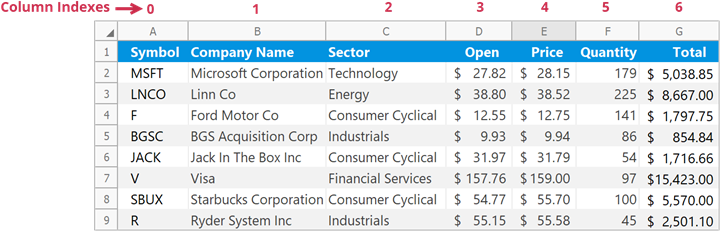#### Obtain the Column with a Given Heading

``````using DevExpress.Spreadsheet;
// ...

// Access the column collection.
ColumnCollection columns = workbook.Worksheets.Columns;

// Access the first column by its heading.
Column firstColumn = columns["A"];
``````

Column headings (“A”, “B”, “C”, etc.) identify each column in a worksheet. Specify column headings in the A1 reference style.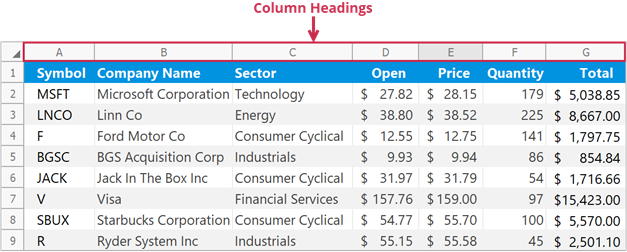### Insert Columns

Use the following methods to insert columns into a worksheet:

Column.Insert
Inserts a column to the left of the current column.
ColumnCollection.Insert
Inserts columns at the specified position.

Note

The number of columns in a worksheet does not change — 16,384. When you add new columns, other columns in the worksheet are shifted to the right, and an equivalent number of columns at the end of the worksheet is removed.

#### Insert a Single Column

``````// Insert column "B".
worksheet.Columns["B"].Insert();

// Insert column "D".
worksheet.Columns.Insert(3);

// Insert column "F".
worksheet.Columns.Insert("F");
``````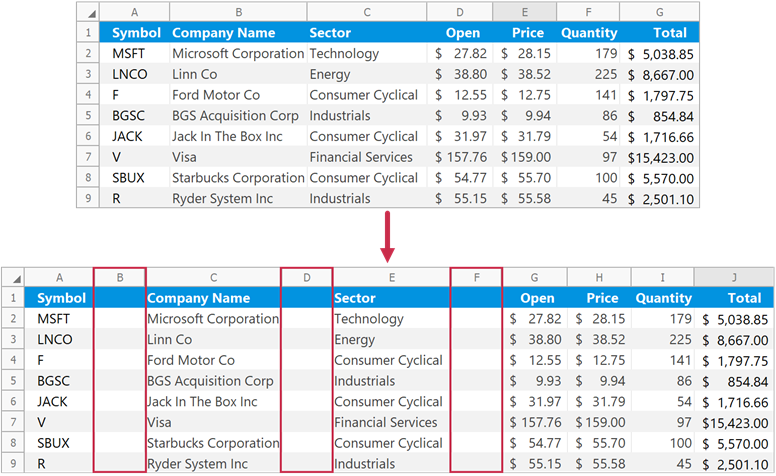#### Insert Multiple Columns

``````// Insert three columns (from column "B" to column "D").
worksheet.Columns.Insert(1, 3);

// Insert two columns starting with column "F".
worksheet.Columns.Insert("F", 2);
``````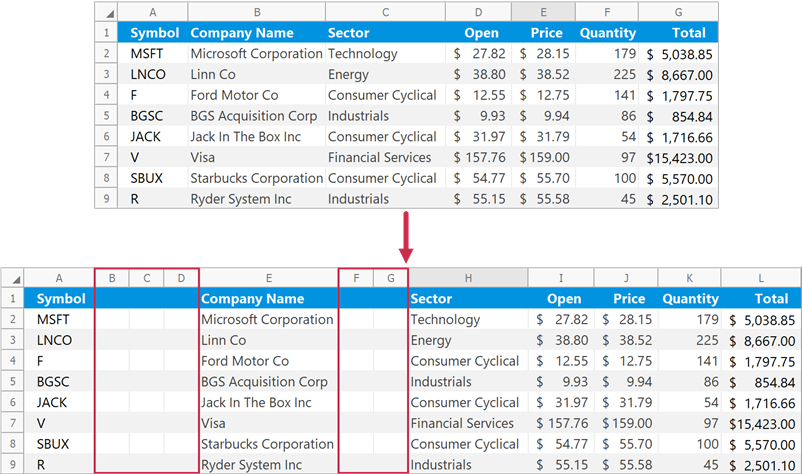#### Insert Columns to the Left of a Specific Cell Range

Use the Worksheet.InsertCells method to insert empty columns to the left of the specified cells. The method inserts the same number of columns as the specified cell range.

``````// Insert three columns to the left of the "B3:D5" cell range.
worksheet.InsertCells(worksheet.Range["B3:D5"], InsertCellsMode.EntireColumn);
``````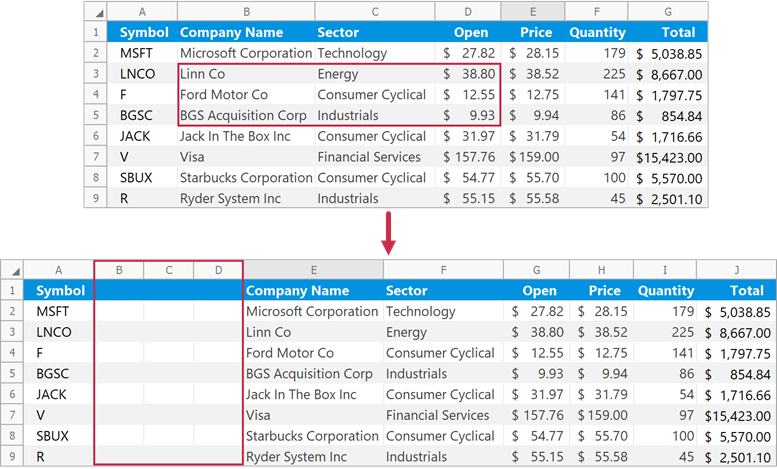#### Format Inserted Columns

Pass a ColumnFormatMode enumeration member to the ColumnCollection.Insert method to specify format options for inserted columns.

The following example inserts two columns (“B” and “C”) and applies the same format as column “A” to these columns:

``````// Insert two columns with the same formatting as the column "A".
worksheet.Columns.Insert("B", 2, ColumnFormatMode.FormatAsPrevious);
``````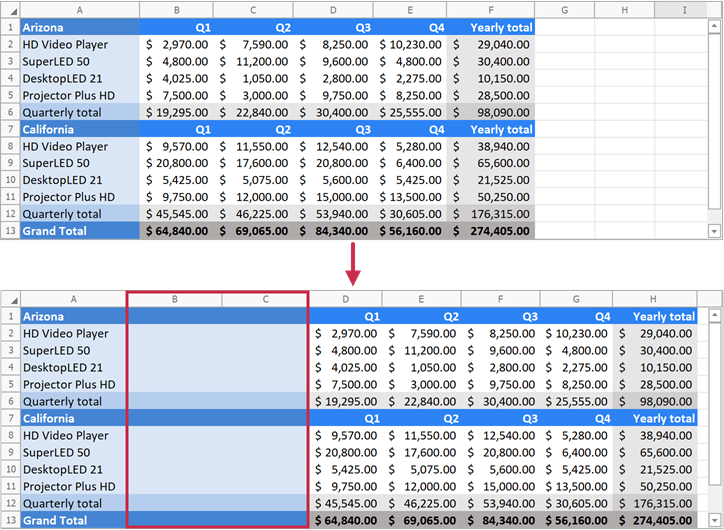### Remove Columns

Use the following methods to delete columns from a worksheet:

Column.Delete
Deletes the current column.
ColumnCollection.Remove
Deletes columns at the specified position or deletes multiple columns at once.

Note

The number of columns in a worksheet does not change — 16,384. When you delete columns, other columns in the worksheet are shifted to the left and the equivalent number of new columns is automatically added to the end of the worksheet’s column collection.

#### Remove a Single Column

``````// Delete column "C".
worksheet.Columns.Remove("C");

// Delete the third column.
worksheet.Columns.Delete();

// Delete the fifth column.
worksheet.Columns.Remove(4);
``````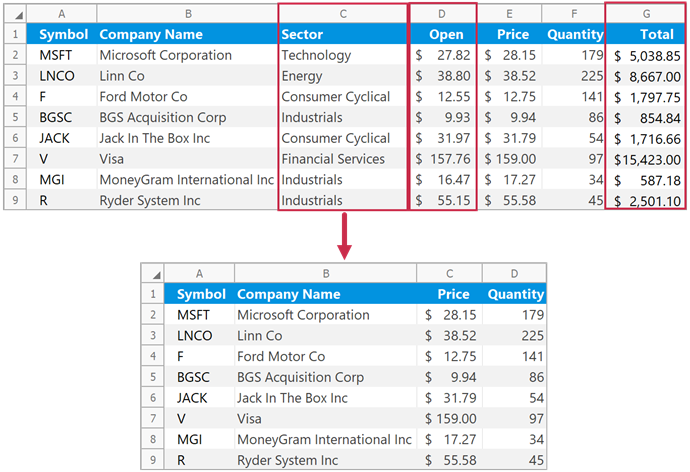#### Remove Multiple Columns

``````// Delete three columns (from column "C" to column "E").
worksheet.Columns.Remove("C", "E");
``````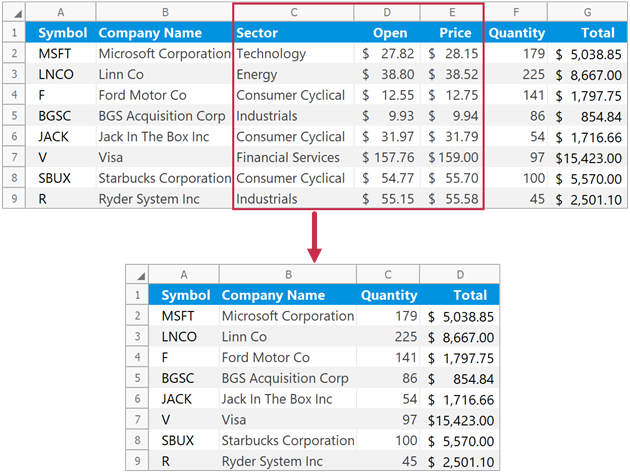#### Remove Columns That Meet a Specific Condition

``````// Specify the condition to remove worksheet columns.
// If text in the first row is "Change in Price", remove the corresponding column.
Func<int, bool> columnRemovalCondition = x => worksheet.Cells[0, x].Value.TextValue == "Change in Price";

// Delete columns that meet the specified condition.
// Check columns "A" through "H".
worksheet.Columns.Remove(0, 7, columnRemovalCondition);
``````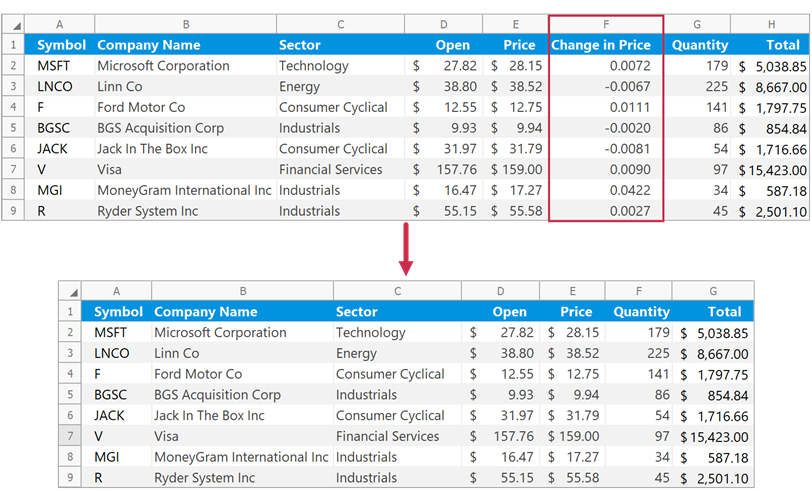#### Remove Columns That Contain Specific Cells

Use the Worksheet.DeleteCells method to delete columns that contain the specified cell range.

``````// Delete a column that contains the "F3" cell.
worksheet.DeleteCells(worksheet.Cells["F3"], DeleteMode.EntireColumn);
``````### Group and Ungroup Columns

Use the ColumnCollection.Group method to group columns.

``````// Group columns "C" through "F" and expand the group.
worksheet.Columns.Group(2, 5, false);

// Group columns "C" and "D" and collapse the group.
worksheet.Columns.Group("C", "D", true);
``````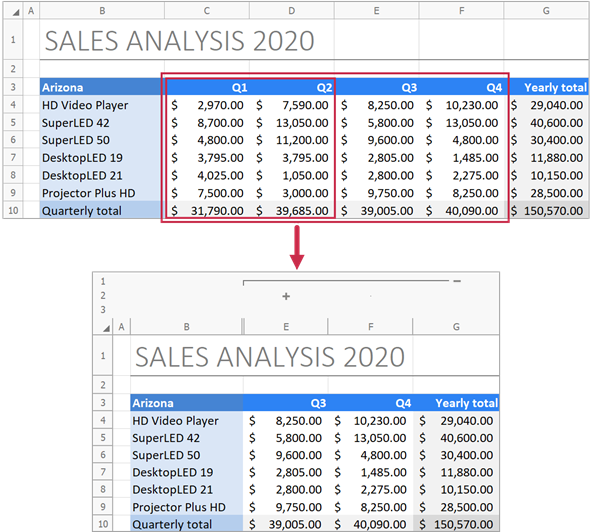Use the ColumnCollection.UnGroup method to ungroup columns.

``````// Ungroup columns "C" through "F".
worksheet.Columns.UnGroup(2, 5, false);

// Ungroup columns "C" and "D" and display collapsed data.
worksheet.Columns.UnGroup("C", "D", true);
``````

#### Expand or Collapse Groups

Use the following methods to collapse or expand column groups in a worksheet:

ColumnCollection.CollapseAllGroups
Collapses all column groups.
ColumnCollection.CollapseGroups
Collapses column groups starting with the specified group level.
ColumnCollection.ExpandAllGroups
Expands all column groups.
``````// Collapse all column groups except the first level group.
worksheet.Columns.CollapseGroups(2);
``````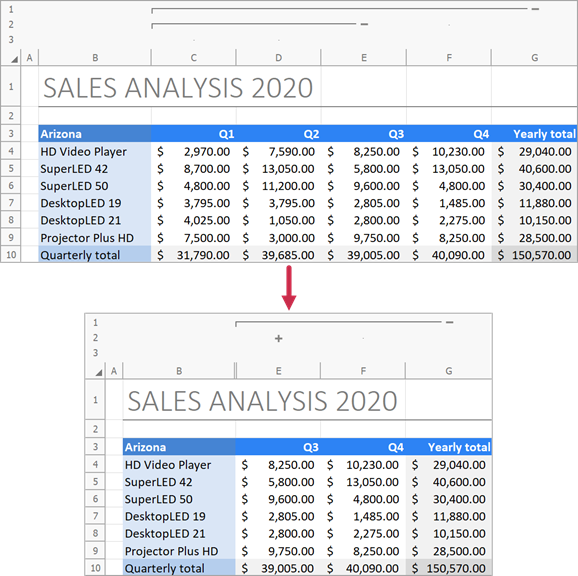### Hide Columns

Use the Column.Visible property or the ColumnCollection.Hide method to hide columns in a worksheet.

``````// Hide column "A".
worksheet.Columns.Visible = false;

// Hide columns "C" through "D".
worksheet.Columns.Hide(2, 3);

// Hide columns "F" through "H".
worksheet.Columns.Hide("F", "H");
``````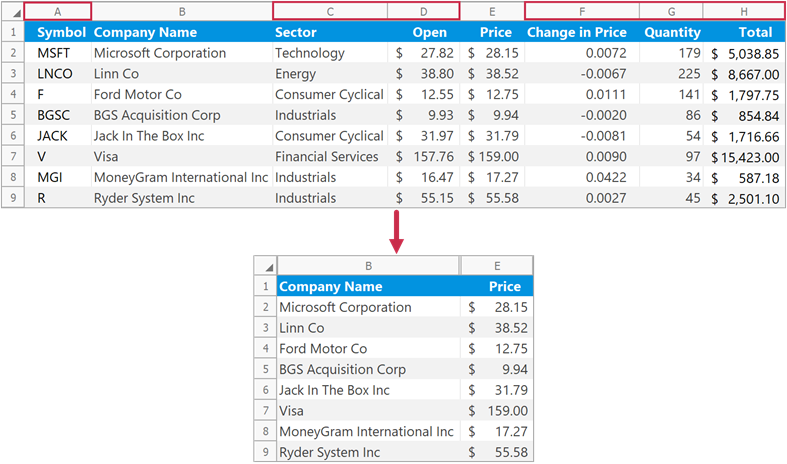Set the Column.Visible property to true to display a column. Use the ColumnCollection.Unhide method to show multiple hidden columns.

``````// Show column "A".
worksheet.Columns.Visible = true;

// Show columns "C" through "D".
worksheet.Columns.Unhide(2, 3);

// Show columns "F" through "H".
worksheet.Columns.Unhide("F", "H");
``````

The following code snippets (auto-collected from DevExpress Examples) contain references to the Columns property.

Note

The algorithm used to collect these code examples remains a work in progress. Accordingly, the links and snippets below may produce inaccurate results. If you encounter an issue with code examples below, please use the feedback form on this page to report the issue.